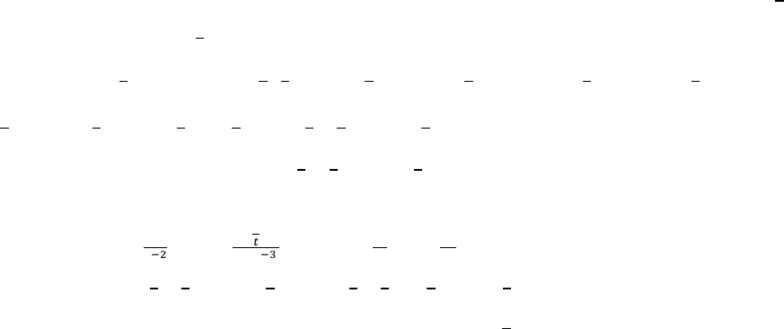Class Notes (1,100,000)
CA (620,000)
UTSG (50,000)
MAT (4,000)
MAT136H1 (900)
all (200)
Lecture

MAT136H1 Lecture Notes - Improper Integral, Asymptote, Antiderivative

Department
Mathematics
Course Code
MAT136H1
Professor
all

Page:
of 17.8 Integration Techniques
Improper Integrals
Question #5 (Medium): Convergent Improper Integral With Infinite Discontinuity
Strategy
When dealing with type improper integrals, the interval usually does not contain infinity but is over
numeric values. It is categorized as improper integral of type because asymptotic value is caught in the
interval. To apply the limit, the asymptotic value needs to be determined first. The value of that makes
the denominator is the value the limit should approach. Once the limit is set, split the interval with
respect to the asymptote, or if one end of the interval is the asymptote, replace that value with variable
. Then disregard the limit and evaluate the integral. Add the anti-derivative to the limit, then plug in the
approaching value to see if it diverges or converges. Sometimes directly plugging in the value cannot be
done. Then l’Hospital’s rule can be applied.
Sample Question
Determine if the integral is convergent or divergent. If convergent, evaluate the integral.


Solution
 does not exist. It is the asymptote. Therefore the beginning of the interval is associated with the
limit. Since approaches the asymptote from the right, limit is set to approach from the right side
with the superscript . Then: 
  

. After setting the limit, focus on solving
the integral alone. The two distinctively different functions can be solved by integration by parts.
Though simplifies with derivative, there is no other way to deal with . So let   , 
,
and   , and
. Integration by parts does not require the interval to be changed. So:




 

  
 
 

 

Then add the limit and solve: 


. Now problem arises because  expression is
still part of the anti-derivative. Take the whole expression , and apply l’Hospital’s rule:

  

 

  

  
 
Therefore, 


  
 
 
Thus, the improper integral is convergent and 
  